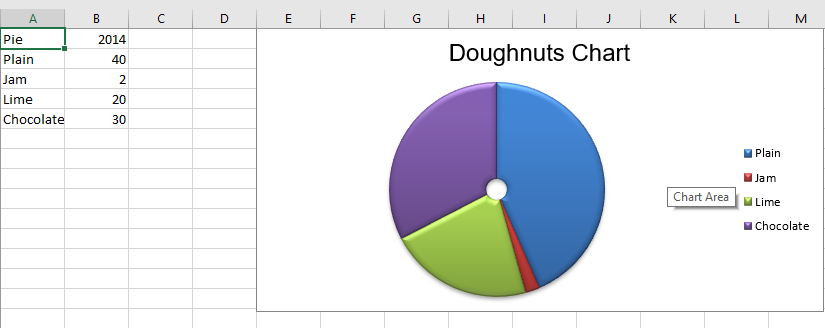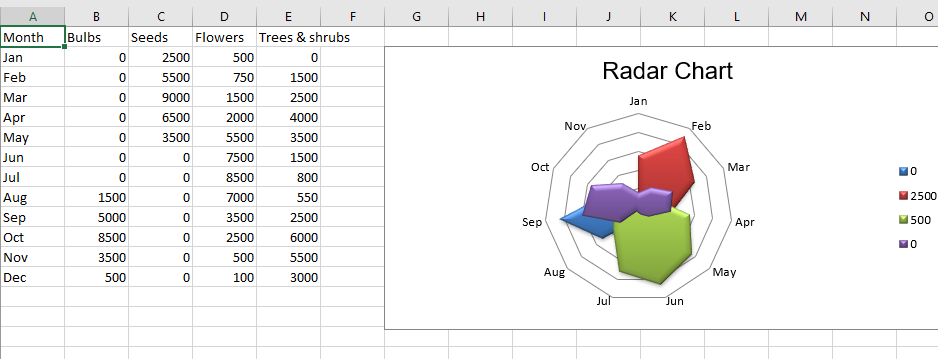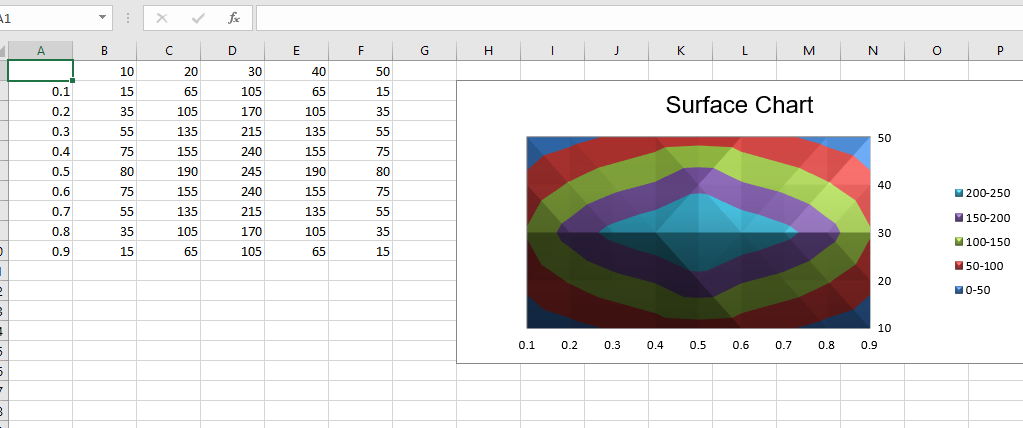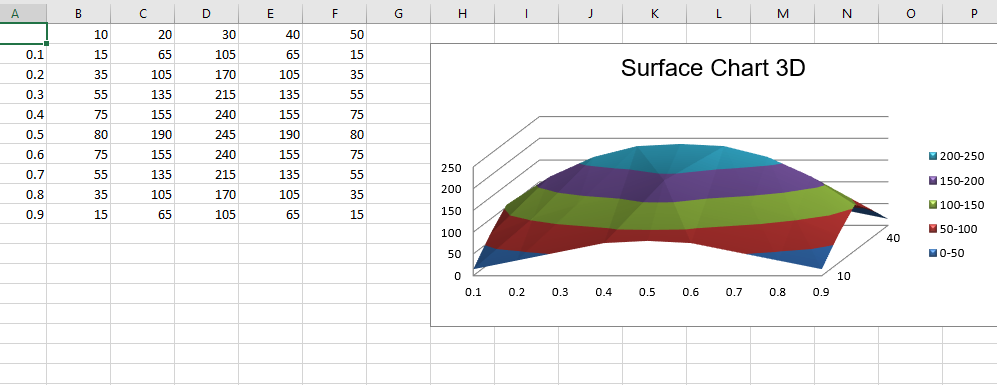GeeksforGeeks App
Open AppBrowser
Continue

# Python | Plotting charts in excel sheet using openpyxl module | Set 3

Prerequisite : Plotting charts in excel sheet using openpyxl module Set – 1 | Set – 2
Openpyxl is a Python library using which one can perform multiple operations on excel files like reading, writing, arithmetic operations and plotting graphs.
Charts are composed of at least one series of one or more data points. Series themselves are comprised of references to cell ranges. Let’s see how to plot Doughnot, Radar, Surface, 3D Surface Chart on an excel sheet using openpyxl.
For plotting the charts on an excel sheet, firstly, create chart object of specific chart class( i.e SurfaceChart, RadarChart etc.). After creating chart objects, insert data in it and lastly, add that chart object in the sheet object. Let’s see how to plot different charts using realtime data.
Code #1 : Plot the Doughnut Chart
Doughnut charts are similar to pie charts except that they use a ring instead of a circle. They can also plot several series of data as concentric rings. For plotting the Doughnut chart on an excel sheet, use DoughnutChart class from openpyxl.chart submodule.

## Python3

 `# import Workbook from openpyxl``from` `openpyxl ``import` `Workbook` `# import DoughnutChart, Reference from openpyxl.chart sub_module .``from` `openpyxl.chart ``import` `DoughnutChart, Reference` `# import DataPoint from openpyxl.chart.series class``from` `openpyxl.chart.series ``import` `DataPoint` `# Call a Workbook() function of openpyxl``# to create a new blank Workbook object``wb ``=` `Workbook()` `# Get workbook active sheet``# from the active attribute.``ws ``=` `wb.active` `# data given``data ``=` `[``    ``[``'Pie'``, ``2014``],``    ``[``'Plain'``, ``40``],``    ``[``'Jam'``, ``2``],``    ``[``'Lime'``, ``20``],``    ``[``'Chocolate'``, ``30``],``]` `# write content of each row in 1st and 2nd``# column of the active sheet respectively .``for` `row ``in` `data:``    ``ws.append(row)` `# Create object of DoughnutChart class``chart ``=` `DoughnutChart()` `# create data for plotting``labels ``=` `Reference(ws, min_col ``=` `1``, min_row ``=` `2``, max_row ``=` `5``)``data ``=` `Reference(ws, min_col ``=` `2``, min_row ``=` `1``, max_row ``=` `5``)` `# adding data to the Doughnut chart object``chart.add_data(data, titles_from_data ``=` `True``)` `# set labels in the chart object``chart.set_categories(labels)` `# set the title of the chart``chart.title ``=` `"Doughnuts Chart"` `# set style of the chart``chart.style ``=` `26` `# add chart to the sheet``# the top-left corner of a chart``# is anchored to cell E1 .``ws.add_chart(chart, ``"E1"``)` `# save the file``wb.save(``"doughnut.xlsx"``)`

Output:Code #2: Plot the Radar Chart
Data that is arranged in columns or rows on a worksheet can be plotted in a radar chart. Radar charts compare the aggregate values of multiple data series. It is effectively a projection of an area chart on a circular x-axis. For plotting the Radar chart on an excel sheet, use RadarChart class from openpyxl.chart submodule.

## Python3

 `# import Workbook from openpyxl``from` `openpyxl ``import` `Workbook` `# import RadarChart, Reference from openpyxl.chart sub_module .``from` `openpyxl.chart ``import` `RadarChart, Reference` `# Call a Workbook() function of openpyxl``# to create a new blank Workbook object``wb ``=` `Workbook()` `# Get workbook active sheet``# from the active attribute.``ws ``=` `wb.active` `# data given``data ``=` `[``    ``[``'Month'``, ``"Bulbs"``, ``"Seeds"``, ``"Flowers"``, ``"Trees & shrubs"``],``    ``[``'Jan'``, ``0``, ``2500``, ``500``, ``0``, ],``    ``[``'Feb'``, ``0``, ``5500``, ``750``, ``1500``],``    ``[``'Mar'``, ``0``, ``9000``, ``1500``, ``2500``],``    ``[``'Apr'``, ``0``, ``6500``, ``2000``, ``4000``],``    ``[``'May'``, ``0``, ``3500``, ``5500``, ``3500``],``    ``[``'Jun'``, ``0``, ``0``, ``7500``, ``1500``],``    ``[``'Jul'``, ``0``, ``0``, ``8500``, ``800``],``    ``[``'Aug'``, ``1500``, ``0``, ``7000``, ``550``],``    ``[``'Sep'``, ``5000``, ``0``, ``3500``, ``2500``],``    ``[``'Oct'``, ``8500``, ``0``, ``2500``, ``6000``],``    ``[``'Nov'``, ``3500``, ``0``, ``500``, ``5500``],``    ``[``'Dec'``, ``500``, ``0``, ``100``, ``3000` `],``]` `# write content of each row in 1st and 2nd``# column of the active sheet respectively .``for` `row ``in` `data:``    ``ws.append(row)` `# Create object of RadarChart class``chart ``=` `RadarChart()` `# filled type of radar chart``chart.``type` `=` `"filled"` `# create data for plotting``labels ``=` `Reference(ws, min_col ``=` `1``, min_row ``=` `2``, max_row ``=` `13``)``data ``=` `Reference(ws, min_col ``=` `2``, max_col ``=` `5``, min_row ``=` `2``, max_row ``=` `13``)` `# adding data to the Radar chart object``chart.add_data(data, titles_from_data ``=` `True``)` `# set labels in the chart object``chart.set_categories(labels)` `# set the title of the chart``chart.title ``=` `"Radar Chart"` `# set style of the chart``chart.style ``=` `26` `# delete y axis from the chart``chart.y_axis.delete ``=` `True` `# add chart to the sheet``# the top-left corner of a chart``# is anchored to cell G2 .``ws.add_chart(chart, ``"G2"``)` `# save the file``wb.save(``"Radar.xlsx"``)`

Output:Code #3 : Plot The Surface Chart
Data that is arranged in columns or rows on a worksheet can be plotted in a surface chart. A surface chart is useful when you want to find optimum combinations between two sets of data. As in a topographic map, colors and patterns indicate areas that are in the same range of values. For plotting the Surface chart on an excel sheet, use SurfaceChart class from openpyxl.chart submodule.

## Python3

 `# import Workbook from openpyxl``from` `openpyxl ``import` `Workbook` `# import SurfaceChart, Reference, Series from openpyxl.chart sub_module .``from` `openpyxl.chart ``import` `SurfaceChart, Reference, Series` `# Call a Workbook() function of openpyxl``# to create a new blank Workbook object``wb ``=` `Workbook()` `# Get workbook active sheet``# from the active attribute.``ws ``=` `wb.active` `# given data``data ``=` `[``    ``[``None``, ``10``, ``20``, ``30``, ``40``, ``50``, ],``    ``[``0.1``, ``15``, ``65``, ``105``, ``65``, ``15``, ],``    ``[``0.2``, ``35``, ``105``, ``170``, ``105``, ``35``, ],``    ``[``0.3``, ``55``, ``135``, ``215``, ``135``, ``55``, ],``    ``[``0.4``, ``75``, ``155``, ``240``, ``155``, ``75``, ],``    ``[``0.5``, ``80``, ``190``, ``245``, ``190``, ``80``, ],``    ``[``0.6``, ``75``, ``155``, ``240``, ``155``, ``75``, ],``    ``[``0.7``, ``55``, ``135``, ``215``, ``135``, ``55``, ],``    ``[``0.8``, ``35``, ``105``, ``170``, ``105``, ``35``, ],``    ``[``0.9``, ``15``, ``65``, ``105``, ``65``, ``15``],``]` `# write content of each row in 1st and 2nd``# column of the active sheet respectively .``for` `row ``in` `data:``    ``ws.append(row)` `# Create object of SurfaceChart class``chart ``=` `SurfaceChart()` `# create data for plotting``labels ``=` `Reference(ws, min_col ``=` `1``, min_row ``=` `2``, max_row ``=` `10``)``data ``=` `Reference(ws, min_col ``=` `2``, max_col ``=` `6``, min_row ``=` `1``, max_row ``=` `10``)` `# adding data to the Surface chart object``chart.add_data(data, titles_from_data ``=` `True``)` `# set labels in the chart object``chart.set_categories(labels)` `# set the title of the chart``chart.title ``=` `"Surface Chart"` `# set style of the chart``chart.style ``=` `26` `# add chart to the sheet``# the top-left corner of a chart``# is anchored to cell H2 .``ws.add_chart(chart, ``"H2"``)` `# save the file``wb.save(``"Surface.xlsx"``)`

Output:Code #4 : Plot the Surface 3D chart
For plotting the 3D Surface chart on an excel sheet, use SurfaceChart3D class from openpyxl.chart submodule.

## Python3

 `# import Workbook from openpyxl``from` `openpyxl ``import` `Workbook` `# import SurfaceChart3D, Reference, Series from openpyxl.chart sub_module .``from` `openpyxl.chart ``import` `SurfaceChart3D, Reference, Series` `# Call a Workbook() function of openpyxl``# to create a new blank Workbook object``wb ``=` `Workbook()` `# Get workbook active sheet``# from the active attribute.``ws ``=` `wb.active` `# given data``data ``=` `[``    ``[``None``, ``10``, ``20``, ``30``, ``40``, ``50``, ],``    ``[``0.1``, ``15``, ``65``, ``105``, ``65``, ``15``, ],``    ``[``0.2``, ``35``, ``105``, ``170``, ``105``, ``35``, ],``    ``[``0.3``, ``55``, ``135``, ``215``, ``135``, ``55``, ],``    ``[``0.4``, ``75``, ``155``, ``240``, ``155``, ``75``, ],``    ``[``0.5``, ``80``, ``190``, ``245``, ``190``, ``80``, ],``    ``[``0.6``, ``75``, ``155``, ``240``, ``155``, ``75``, ],``    ``[``0.7``, ``55``, ``135``, ``215``, ``135``, ``55``, ],``    ``[``0.8``, ``35``, ``105``, ``170``, ``105``, ``35``, ],``    ``[``0.9``, ``15``, ``65``, ``105``, ``65``, ``15``],``]` `# write content of each row in 1st and 2nd``# column of the active sheet respectively .``for` `row ``in` `data:``    ``ws.append(row)` `# Create object of SurfaceChart3D class``chart ``=` `SurfaceChart3D()` `# create data for plotting``labels ``=` `Reference(ws, min_col ``=` `1``, min_row ``=` `2``, max_row ``=` `10``)``data ``=` `Reference(ws, min_col ``=` `2``, max_col ``=` `6``, min_row ``=` `1``, max_row ``=` `10``)` `# adding data to the Surface chart 3D object``chart.add_data(data, titles_from_data ``=` `True``)` `# set labels in the chart object``chart.set_categories(labels)` `# set the title of the chart``chart.title ``=` `"Surface Chart 3D"` `# set style of the chart``chart.style ``=` `26` `# add chart to the sheet``# the top-left corner of a chart``# is anchored to cell H2 .``ws.add_chart(chart, ``"H2"``)` `# save the file``wb.save(``"Surface3D.xlsx"``)`

Output :My Personal Notes arrow_drop_up